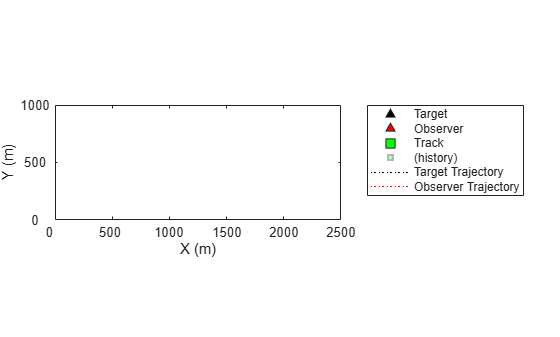initcvmscekf

Constant velocity trackingMSCEKF initialization

Description

example

mscekf = initcvmscekf(detection) initializes a trackingMSCEKF class (extended Kalman filter for tracking in modified spherical coordinates) based on information provided in an objectDetection object, detection. The function assumes a target range of 3e4 units and a range-covariance of 1e10 units2.

The trackingMSCEKF object can be used with trackers for tracking targets with angle-only measurements from a single observer.

example

mscekf = initcvmscekf(detection,rangeEstimation) allows specifying the range information to the filter. The rangeEstimation variable is a two-element vector, where the first element specifies the range of the target, and the second element specifies the standard deviation in range.

Examples

collapse all

Create an angle-only detection.

detection = objectDetection(0,[30;20],'MeasurementParameters',...
struct('Frame','Spherical','HasRange',false));

Use initcvmscekf to create a trackingMSCEKF filter initialized using the angle-only detection.

filter = initcvmscekf(detection)
filter =
trackingMSCEKF with properties:

State: [6x1 double]
StateCovariance: [6x6 double]

StateTransitionFcn: @constvelmsc
StateTransitionJacobianFcn: @constvelmscjac
ProcessNoise: [3x3 double]
ObserverInput: [3x1 double]

MeasurementFcn: @cvmeasmsc
MeasurementJacobianFcn: @cvmeasmscjac
MeasurementNoise: [2x2 double]

Create measurement parameters for subsequent rotation.

measParamSensorToPlat = struct('Frame','Spherical','HasRange',false,...
'Orientation',rotmat(quaternion([0 0 30],'rotvecd'),'frame'))
measParamSensorToPlat = struct with fields:
Frame: 'Spherical'
HasRange: 0
Orientation: [3x3 double]

measParamPlatToScenario = struct('Frame','Rectangular','HasRange',false,...
'Orientation',rotmat(quaternion([30 0 0],'rotvecd'),'frame'))
measParamPlatToScenario = struct with fields:
Frame: 'Rectangular'
HasRange: 0
Orientation: [3x3 double]

measParam = [measParamSensorToPlat;measParamPlatToScenario];
detection = objectDetection(0,[30;20],'MeasurementParameters',measParam);

Initialize a filter.

filter = initcvmscekf(detection);

Check that filter's measurement is same as detection.

cvmeasmsc(filter.State,measParam)
ans = 2×1

30.0000
20.0000

Consider a scenario when the target is moving at a constant velocity along and the observer is moving at a constant acceleration. Define target's initial state using a constant velocity model.

tgtState = [2000;-3;500;-5;0;0];

Define observer's initial state using a constant acceleration model.

observerState = [0;2;0;490;-10;0.2;0;0;0];

Create a trackerGNN object to use with initcvmscekf with some prior information about range and range-covariance.

range = 1000;
rangeStdDev = 1e3;
rangeEstimate = [range rangeStdDev];
tracker = trackerGNN('FilterInitializationFcn',@(det)initcvmscekf(det,rangeEstimate));

Simulate synthetic data by using measurement models. Get az and el information using the cvmeas function.

syntheticParams = struct('Frame','Spherical','HasRange',false,...
'OriginPosition',observerState(1:3:end));
meas = cvmeas(tgtState,syntheticParams);

Create an angle-only objectDetection to simulate synthetic detection.

detection = objectDetection(0,meas,'MeasurementParameters',...
struct('Frame','Spherical','HasRange',false),'MeasurementNoise',0.033*eye(2));

Create trackPlotter and platformPlotter to visualize the scenario.

tp = theaterPlot('XLimits',[0 2500],'YLimits',[0 1000]);
targetPlotter = platformPlotter(tp,'DisplayName','Target','MarkerFaceColor','k');
observerPlotter = platformPlotter(tp,'DisplayName', 'Observer','MarkerFaceColor','r');
trkPlotter = trackPlotter(tp,'DisplayName','Track','MarkerFaceColor','g','HistoryDepth',50);
tgtTrajPlotter = trajectoryPlotter(tp,'DisplayName','Target Trajectory','Color','k');
obsTrajPlotter = trajectoryPlotter(tp,'DisplayName','Observer Trajectory','Color','r');Run the tracker.

time = 0; dT = 0.1;
tgtPoses = [];
obsPoses = [];
while time < 50
[confTracks,tentTracks,allTracks] = tracker(detection,time);
for i = 1:numel(allTracks)
setTrackFilterProperties(tracker,allTracks(i).TrackID,'ObserverInput',observerState(3:3:end));
end

% Update synthetic detection.
observerState = constacc(observerState,dT);
tgtState = constvel(tgtState,dT);
syntheticParams.OriginPosition = observerState(1:3:end);
detection.Measurement = cvmeas(tgtState,syntheticParams);
time = time + dT;
detection.Time = time;

% Update plots
tgtPoses = [tgtPoses;tgtState(1:2:end)']; %#ok
obsPoses = [obsPoses;observerState(1:3:end)']; %#ok
targetPlotter.plotPlatform(tgtState(1:2:end)');
observerPlotter.plotPlatform(observerState(1:3:end)');
tgtTrajPlotter.plotTrajectory({tgtPoses});
obsTrajPlotter.plotTrajectory({obsPoses});
% Plot the first track as there are no false alarms, this should be
% the target.
% Get positions from the MSC state of the track.
cartState = cvmeasmsc(allTracks(i).State,'rectangular') + observerState(1:3:end);
trkPlotter.plotTrack(cartState');
endInput Arguments

collapse all

Detection report, specified as an objectDetection object.

Example: detection = objectDetection(0,[1;4.5;3],'MeasurementNoise', [1.0 0 0; 0 2.0 0; 0 0 1.5])

Range information, specified as a two-element vector, where the first element specifies the range of the target, and the second element specifies the standard deviation in range.

Data Types: single | double

Output Arguments

collapse all

Constant velocity tracking extended Kalman filter in an MSC frame, returned as a trackingMSCEKF object.

Algorithms

• The function configures the filter with process noise assuming a unit target acceleration standard deviation.

• The function configures the covariance of the state in an MSC frame by using a linear transformation of covariance in a Cartesian frame.

• You can use this function as the FilterInitializationFcn property of trackerTOMHT and trackerGNN System objects.

• The function initializes the ObserverInput of the trackingMSCEKF class with zero observer acceleration in all directions. You must use the setTrackFilterProperties function of the trackers to update the ObserverInput.